# Union of Sets

In previous lessons, we used Venn diagrams to represent relationships between sets. Let's look at example 1 below.

Example 1: In Greenville Middle School, two classes will be merged into one in order to reduce costs. If the students in Band and Chorus are combined into one new class, then which students will be in that class?

Given: Given= {Sam, Kyesha, Derek, Lorrie, Robin, Raúl, Shirley, Nathan, Chris, Dana},  Band = {Sam, Lorrie, Raúl, Derek} and Chorus = {Robin, Derek, Kyesha}, find Band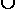Chorus.

Analysis: This problem is asking us to find the union of Band and Chorus. To solve this problem, we must list all students who are in Band, in Chorus, or in both.

Solution:Explanation:

BandChorus = {Sam, Lorrie, Raúl, Derek, Kyesha, Robin}

Note that Derek is in both sets, and therefore, in their intersection. However, Derek is only written once in the union.

Definition: The union of two sets A and B, is the set of elements which are in A or in or in both. It is denoted by AB, and is read "A union B".

Let's compare union and intersection.

 Union Intersection written as AB AB read as A union B A intersect B meaning of A or B or both A and B how to find combine all elements find elements in common to both

A union is often thought of as a marriage. We use "and" for intersection" and "or" for union. Let's look at some more examples of the union of two sets.

Example 2: Let= {counting numbers}, P = {multiples of 3 less than 20} and Q = {even numbers less than 20}. Draw and label a Venn diagram to show the union of P and Q.

Analysis: Shade elements which are in P or in Q or in both. The shaded region in the Venn diagram below indicates PQ.

Solution: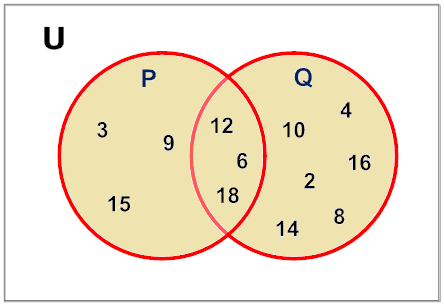Notation: PQ = {2, 3, 4, 6, 8, 9, 10, 12, 14, 15, 16, 18}

Note that the elements 6, 12 and 18 are in both sets (i.e., in the intersection of P and Q). However, these elements are only written once in the union.

Example 3: Let=  { k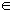| 1   k   10 }, X = {1, 6, 9} and Y = {1, 3, 5, 6, 8, 9}. Draw and label a Venn diagram to showY.

Analysis: X is a subset of Y. So all elements in X are also contained in Y.Explanation: XY = {1, 3, 5, 6, 8, 9}, which is equal to the set Y.

In example 3, since X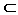Y , we get that XY = Y. This relationship is defined below.Another way to define the union of two sets is as follows:

AB = {x | xA or xB}

Example 4: Let= {animals}, A = {10 dogs} and B = {20 cats}. Draw and label a Venn diagram to show the AB.

Analysis: These sets are disjoint, and have no elements in common. Thus,is all the elements in A and all the elements in B.Explanation:B = {10 dogs, 20 cats}

Example 4 is a straight forward union of two sets. Disjoint sets have no elements in common. Therefore the union of A and B has no common elements. Since it is not possible that dogs in A are also cats in B, we are sure that the numbers of elements in the union is sum of all dogs in A and all cats in B. Thus, the union results from the addition of all elements in both sets.

The union of two sets A and B, is the set of elements which are in A or in or in both. It is denoted by AB, and is read "A union B". The formal definition of union is shown below:

AB = {x | xA or xB}

Exercises

 1. Given= {apples, oranges, bananas, pears} and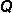= {oranges, pears, grapes}, what is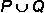? PQ = Ø PQ = {apples, oranges, bananas, pears} PQ = {apples, oranges, bananas, pears, grapes} None of the above. RESULTS BOX:
 2. Given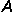= {prime numbers} and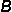= {composite numbers}, what is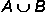? AB = {whole numbers} AB = {counting numbers > 1} AB = {imaginary numbers} All of the above. RESULTS BOX:
 3. Given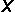= {1, 4, 16} and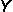= {1, 4, 9, 16, 25, 36}, what is? XY = {1, 4, 9, 16, 25, 36} XY = {1, 4, 16} XY = Ø None of the above. RESULTS BOX:
 4. Given= {vowels} and= {consonants}, what is? PQ = Ø PQ = {a, e, i, o, u} PQ = {consonants} PQ = {The English alphabet} RESULTS BOX:
 5. Which of the following is true about the Venn diagram below?B' = {2, 3, 9} B = {0, 1, 4, 5, 6, 7, 8}= {0, 1, 2, 3, 4, 5, 6, 7, 8, 9} All of the above. RESULTS BOX: